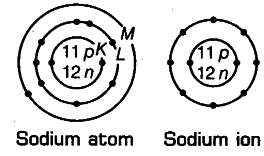# Show diagramatically the electron distributions in a sodium atom

Show diagramatically the electron distributions in a sodium atom and a sodium ion and also give their atomic number.

Atomic number of sodium (Z) =11 Mass number of sodium (A) = 23
Number of protons in the nucleus = 11 Number of neutrons in the nucleus =23-11 = 12
Number of electrons = 11
Electronic configuration of Na-atom = 2, 8, 1(K,L,M)
Na+ ion is formed from s,odium atom by loss of an electron (present in the outermost shell). Hence, its
electronic configuration is 2, 8(K,L). However, number of protons and neutrons remains the same.